# Example Code

## Overview

This step-by-step demo is designed to examine the relationship between the M-ary of an FSK transmitter and the resulting frequency domain. Frequency Shift Keying (FSK) is a digital modulation scheme in which digital information is transmitted through changes in a carrier sinusoid’s frequency. In digital communication, there are several types of modulation schemes which build on the same fundamental concepts of amplitude, frequency, or phase modulation. Here, information is transmitted though shifts in frequency. In addition, as the term “Shift Keying” implies, changes in frequency are used to represent discrete digital values. Other variations of FSK include prefixes that indicate symbol number (B = 2, Q = 4, M = any). However, you can often omit the acronym prefix if M = 2. Therefore, BFSK is synonymous with FSK. FSK is a simple modulation paradigm used in RFID, cordless phones, caller ID, and many other applications.

### FSK Coherency:

Frequency shift keying can be divided into two types, coherent and non coherent.  In coherent forms of FSK, there is no phase discontinuity in the output signal. FSK modulation formats generate modulated waveforms that are strictly real values, and thus tend not to share common features with quadrature amplitude modulation (QAM) schemes.  In noncoherent forms of FSK, the instantaneous frequency is shifted between two discrete values.  In BPSK, these are referred to as the “mark” and “space” frequencies.  In this demonstration, we will illustrate noncoherent because it can be more easily represented in the frequency domain.

### Exercise:

1. First open the FSK Step by Step template and switch to the block diagram, shown below.  As you can see, several controls, constants, and indicators have already been created and organized for ease of programming.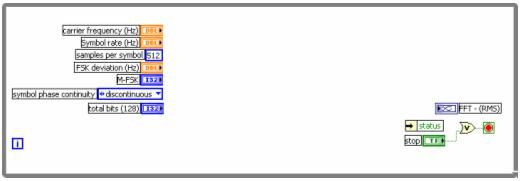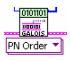2. Next, browse to the modulation palette and place an “MT Bit Generation” VI the block diagram and wire the “total bits(128)” control to the total bits input.  This VI will generate a digital bit stream that will later be modulated using FSK modulation.3. Place a “Generate System Parameters” VI on the block diagram and select the polymorphic instance FSK (M).  Wire the “samples per symbol” and “symbol phase continuity” constants to the inputs of the same name on Generate System Parameters.   Next, wire the “FSK deviation (Hz)” and “M-FSK” controls to the appropriate inputs as well.  Finally, connect the “error out” terminal of the previous VI to the “error in” input of Generate System parameters.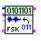4. Place a “Modulate FSK” VI on the block diagram and wire in the system parameters and error in from the previous VI.  Also wire “bitstream” output MT Generate Bits to the bitstream input of Modulate FSK.5. Insert “Upconversion.vi” and wire the input “carrier frequency” to the appropriate control.  The “complex waveform” input should be wired from the output of “Modulate FSK.vi.”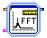6. Next, add the “Spectral Measurements Express VI” by browsing to: Express >> Signal Analysis.  We will use the default settings so select “OK” to close the dialog box and the Express VI will now appear on the block diagram.  To save space, right click on this and select “View as Icon” to save space on the block diagram.7. After that, wire the “Signals” input of the Spectral Measurements VI to the “Waveform” output of the previous VI.  Next, wire the “FFT (RMS) output to the “FFT - (RMS)” graph indicator on the block diagram.  The block diagram should now appear as the diagram shown below.### Demonstration:

Next, we will examine various parameters of an FSK transmitter and observe their affects in the frequency domain.  Most notably, we will change characteristics such as: M-ary, deviation frequency, and symbol rate to observe the effects upon the frequency domain of the signal.

1. First, click on the LabVIEW run button to begin executing the program just created.  Note in the FFT(RMS) graph that all of the power in the frequency domain is centered at 5 MHz.  In addition, four peaks are visible and they are spaced by approximately 650 MHz.  These peaks correspond to the four frequencies being used in transition.  Note that “4” has been selected in the “M-FSK” control.  This is referred to as the M-ary of the modulation scheme and indicates that data is transmitted by adjusting the frequency so that it is one of 4 values.2. Next, we will explore the affect of M-ary on the frequency domain.  Adjust the M-FSK value to all possible values (2,4,8) and observe the affect in the frequency domain.  As you can see, the number of peaks corresponds to the number of frequencies chosen for the M-ary.  You will notice that when M-FSK = 8, that that each of the peaks interfere with one another.  This occurs because the frequencies are not appropriately spaced.  This issue is addressed in the next step.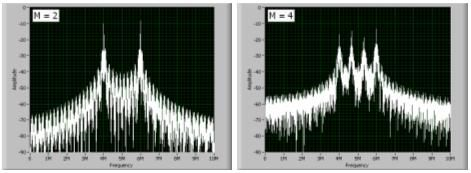3. FSK deviation determines the spacing of each of these peaks.  More specifically, it defines the difference, in frequency, between the carrier frequency and the outermost frequency band.  So far, we have used 1 MHz for this value.  However, when 8-MSK is chosen, the bandwidth of each channel is too wide for the frequency deviation.  Thus, the channels are overlapping.  Thus, we can change the FSK deviation (Hz) control to accommodate more channels.  Experiment with this value and observe the results in the frequency domain.  Below, we show the frequency spectrum with frequency deviations of 1.25 MHz and 3 MHz.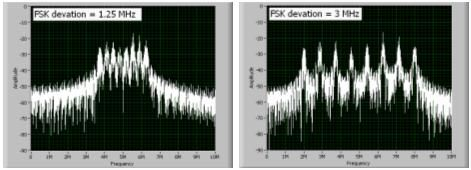4. The final parameter illustrated in this demonstration is the affect of symbol rate on channel bandwidth.  While this concept is more thoroughly explored in (Link to Channel Bandwidth), we will touch on it briefly here.  While the VI is running, slowly reduce the bandwidth from 200 kHz to 50 kHz.  You will observe that each of the frequency peaks become narrower as the symbol rate decreases.  As a result, it is possible to use a smaller portion of the frequency domain.  In fact, even with eight distinct frequencies, we can reduce our frequency deviation and move each of the peaks closer together without overlapping.  However, symbol rate is directly proportional to data throughput.  Thus, in a real-world communications system, there are tradeoffs between bandwidth and system performance.  Below, we show eight distinct peaks from our modulated waveform.### Conclusion:

As this demonstration illustrates, FSK involves the transmission of digital information through variations of a carrier’s frequency.  In addition, it illustrates the relationship between carrier frequency, M-ary, frequency deviation, and symbol rate.

Example code from the Example Code Exchange in the NI Community is licensed with the MIT license.